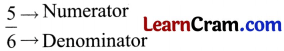# DAV Class 3 Maths Chapter 11 Worksheet 2 Solutions

The DAV Maths Book Class 3 Solutions and DAV Class 3 Maths Chapter 11 Worksheet 2 Solutions of Fractions offer comprehensive answers to textbook questions.

## DAV Class 3 Maths Ch 11 WS 2 Solutions

Question 1.
Write the numerator of the following fractions:

(a) $$\frac{2}{3}$$ ______
Solution:
2

(b) $$\frac{1}{9}$$ ______
Solution:
1

(c) $$\frac{13}{15}$$ ______
Solution:
13

(d) $$\frac{4}{6}$$ ______
Solution:
4

(e) $$\frac{7}{15}$$ ______
Solution:
7

(f) $$\frac{6}{10}$$ ______
Solution:
6Question 2.
Write the denominators of the following fractions:

(a) $$\frac{2}{3}$$ ______
Solution:
3

(b) $$\frac{1}{9}$$ ______
Solution:
9

(c) $$\frac{13}{15}$$ ______
Solution:
15

(d) $$\frac{4}{6}$$ ______
Solution:
6

(e) $$\frac{7}{15}$$ ______
Solution:
15

(f) $$\frac{6}{10}$$ ______
Solution:
10Question 3.
Write the fractions for the following. The first one is done for you.

(a) Numerator = 2, Denominator = 7
Solution:
$$\frac{2}{7}$$

(b) Numerator = 5, Denominator = 9
Solution:
$$\frac{5}{9}$$

(c) Numerator = 3, Denominator = 13
Solution:
$$\frac{3}{13}$$

(d) Denominator = 14, Numerator = 8
Solution:
$$\frac{14}{8}$$

(e) Denominator = 8, Numerator = 3
Solution:
$$\frac{8}{3}$$### DAV Class 3 Maths Chapter 11 Worksheet 2 NotesTop number of fraction is called Numerator and bottom number of fraction is called Denominator.